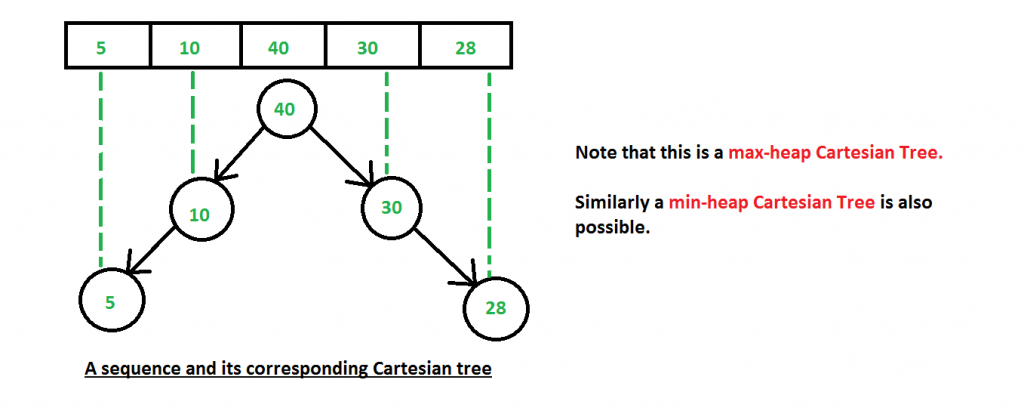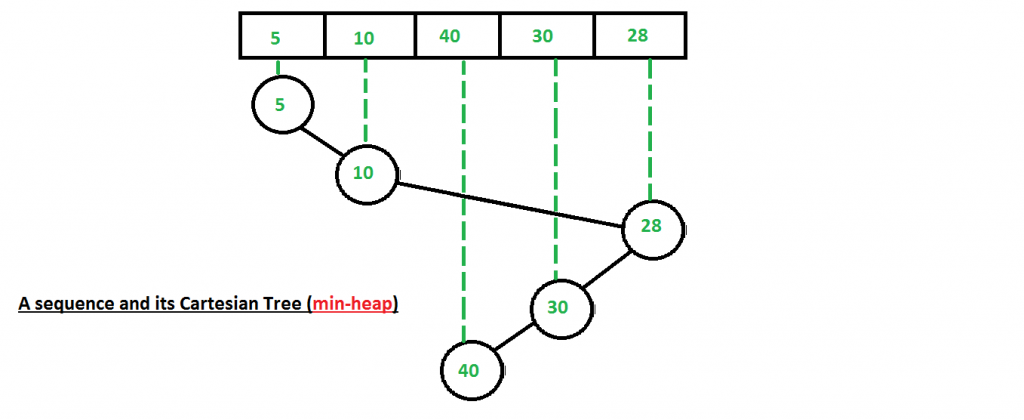# Cartesian Tree

• Difficulty Level : Hard
• Last Updated : 18 Feb, 2022

A Cartesian tree is a tree data structure created from a set of data that obeys the  following structural invariants:

1. The tree obeys in the min (or max) heap property – each node is less (or greater) than its children.
2. An inorder traversal of the nodes yields the values in the same order in which they appear in the initial sequence.

Suppose we have an input array- {5,10,40,30,28}. Then the max-heap Cartesian Tree would be.A min-heap Cartesian Tree of the above input array will be-Note:

1. Cartesian Tree is not a height-balanced tree.
2. Cartesian tree of a sequence of distinct numbers is always unique.

Cartesian tree of a sequence of distinct numbers is always unique.
We will prove this using induction. As a base case, empty tree is always unique. For the inductive case, assume that for all trees containing n’ < n elements, there is a unique Cartesian tree for each sequence of n’ nodes. Now take any sequence of n elements. Because a Cartesian tree is a min-heap, the smallest element of the sequence must be the root of the Cartesian tree. Because an inorder traversal of the elements must yield the input sequence, we know that all nodes to the left of the min element must be in its left subtree and similarly for the nodes to the right. Since the left and right subtree are both Cartesian trees with at most n-1 elements in them (since the min element is at the root), by the induction hypothesis there is a unique Cartesian tree that could be the left or right subtree. Since all our decisions were forced, we end up with a unique tree, completing the induction.
How to construct Cartesian Tree?
A O(n2) solution for construction of Cartesian Tree is discussed here (Note that the above program here constructs the “special binary tree” (which is nothing but a Cartesian tree)
A O(nlogn) Algorithm :
It’s possible to build a Cartesian tree from a sequence of data in O(NlogN) time on average. Beginning with the empty tree,
Scan the given sequence from left to right adding new nodes as follows:

1. Position the node as the right child of the rightmost node.
2. Scan upward from the node’s parent up to the root of the tree until a node is found whose value is greater than the current value.
3. If such a node is found, set its right child to be the new node, and set the new node’s left child to be the previous right child.
4. If no such node is found, set the new child to be the root, and set the new node’s left child to be the previous tree.

## CPP

 `// A O(n) C++ program to construct cartesian tree``// from a given array``#include` `/* A binary tree node has data, pointer to left``   ``child and a pointer to right child */``struct` `Node``{``    ``int` `data;``    ``Node *left, *right;``};` `/* This function is here just to test buildTree() */``void` `printInorder (Node* node)``{``    ``if` `(node == NULL)``        ``return``;``    ``printInorder (node->left);``    ``cout << node->data << ``" "``;``    ``printInorder (node->right);``}` `// Recursively construct subtree under given root using``// leftChild[] and rightchild``Node * buildCartesianTreeUtil (``int` `root, ``int` `arr[],``          ``int` `parent[], ``int` `leftchild[], ``int` `rightchild[])``{``    ``if` `(root == -1)``        ``return` `NULL;` `    ``// Create a new node with root's data``    ``Node *temp = ``new` `Node;``    ``temp->data = arr[root] ;` `    ``// Recursively construct left and right subtrees``    ``temp->left = buildCartesianTreeUtil( leftchild[root],``                    ``arr, parent, leftchild, rightchild );``    ``temp->right = buildCartesianTreeUtil( rightchild[root],``                    ``arr, parent, leftchild, rightchild );` `    ``return` `temp ;``}` `// A function to create the Cartesian Tree in O(N) time``Node * buildCartesianTree (``int` `arr[], ``int` `n)``{``    ``// Arrays to hold the index of parent, left-child,``    ``// right-child of each number in the input array``    ``int` `parent[n],leftchild[n],rightchild[n];` `    ``// Initialize all array values as -1``    ``memset``(parent, -1, ``sizeof``(parent));``    ``memset``(leftchild, -1, ``sizeof``(leftchild));``    ``memset``(rightchild, -1, ``sizeof``(rightchild));` `    ``// 'root' and 'last' stores the index of the root and the``    ``// last processed of the Cartesian Tree.``    ``// Initially we take root of the Cartesian Tree as the``    ``// first element of the input array. This can change``    ``// according to the algorithm``    ``int` `root = 0, last;` `    ``// Starting from the second element of the input array``    ``// to the last on scan across the elements, adding them``    ``// one at a time.``    ``for` `(``int` `i=1; i<=n-1; i++)``    ``{``        ``last = i-1;``        ``rightchild[i] = -1;` `        ``// Scan upward from the node's parent up to``        ``// the root of the tree until a node is found``        ``// whose value is greater than the current one``        ``// This is the same as Step 2 mentioned in the``        ``// algorithm``        ``while` `(arr[last] <= arr[i] && last != root)``            ``last = parent[last];` `        ``// arr[i] is the largest element yet; make it``        ``// new root``        ``if` `(arr[last] <= arr[i])``        ``{``            ``parent[root] = i;``            ``leftchild[i] = root;``            ``root = i;``        ``}` `        ``// Just insert it``        ``else` `if` `(rightchild[last] == -1)``        ``{``            ``rightchild[last] = i;``            ``parent[i] = last;``            ``leftchild[i] = -1;``        ``}` `        ``// Reconfigure links``        ``else``        ``{``            ``parent[rightchild[last]] = i;``            ``leftchild[i] = rightchild[last];``            ``rightchild[last] = i;``            ``parent[i] = last;``        ``}` `    ``}` `    ``// Since the root of the Cartesian Tree has no``    ``// parent, so we assign it -1``    ``parent[root] = -1;` `    ``return` `(buildCartesianTreeUtil (root, arr, parent,``                                    ``leftchild, rightchild));``}` `/* Driver program to test above functions */``int` `main()``{``    ``/* Assume that inorder traversal of following tree``       ``is given``         ``40``       ``/   \``      ``10     30``     ``/         \``    ``5          28 */` `    ``int` `arr[] = {5, 10, 40, 30, 28};``    ``int` `n = ``sizeof``(arr)/``sizeof``(arr);` `    ``Node *root = buildCartesianTree(arr, n);` `    ``/* Let us test the built tree by printing Inorder``       ``traversal */``    ``printf``(``"Inorder traversal of the constructed tree : \n"``);``    ``printInorder(root);` `    ``return``(0);``}`

## Java

 `// A O(n) Java program to construct cartesian tree``// from a given array` `/* A binary tree node has data, pointer to left``child and a pointer to right child */``class` `GFG``{``static` `class` `Node``{``    ``int` `data;``    ``Node left, right;``};` `/* This function is here just to test buildTree() */``static` `void` `printInorder (Node node)``{``    ``if` `(node == ``null``)``        ``return``;``    ``printInorder (node.left);``    ``System.out.print(node.data + ``" "``);``    ``printInorder (node.right);``}` `// Recursively construct subtree under given root using``// leftChild[] and rightchild``static` `Node buildCartesianTreeUtil (``int` `root, ``int` `arr[],``        ``int` `parent[], ``int` `leftchild[], ``int` `rightchild[])``{``    ``if` `(root == -``1``)``        ``return` `null``;` `    ``// Create a new node with root's data``    ``Node temp = ``new` `Node();``    ``temp.data = arr[root] ;` `    ``// Recursively construct left and right subtrees``    ``temp.left = buildCartesianTreeUtil( leftchild[root],``                    ``arr, parent, leftchild, rightchild );``    ``temp.right = buildCartesianTreeUtil( rightchild[root],``                    ``arr, parent, leftchild, rightchild );` `    ``return` `temp ;``}` `// A function to create the Cartesian Tree in O(N) time``static` `Node buildCartesianTree (``int` `arr[], ``int` `n)``{``    ``// Arrays to hold the index of parent, left-child,``    ``// right-child of each number in the input array``    ``int` `[]parent = ``new` `int``[n];``    ``int` `[]leftchild = ``new` `int``[n];``    ``int` `[]rightchild = ``new` `int``[n];` `    ``// Initialize all array values as -1``    ``memset(parent, -``1``);``    ``memset(leftchild, -``1``);``    ``memset(rightchild, -``1``);` `    ``// 'root' and 'last' stores the index of the root and the``    ``// last processed of the Cartesian Tree.``    ``// Initially we take root of the Cartesian Tree as the``    ``// first element of the input array. This can change``    ``// according to the algorithm``    ``int` `root = ``0``, last;` `    ``// Starting from the second element of the input array``    ``// to the last on scan across the elements, adding them``    ``// one at a time.``    ``for` `(``int` `i = ``1``; i <= n - ``1``; i++)``    ``{``        ``last = i - ``1``;``        ``rightchild[i] = -``1``;` `        ``// Scan upward from the node's parent up to``        ``// the root of the tree until a node is found``        ``// whose value is greater than the current one``        ``// This is the same as Step 2 mentioned in the``        ``// algorithm``        ``while` `(arr[last] <= arr[i] && last != root)``            ``last = parent[last];` `        ``// arr[i] is the largest element yet; make it``        ``// new root``        ``if` `(arr[last] <= arr[i])``        ``{``            ``parent[root] = i;``            ``leftchild[i] = root;``            ``root = i;``        ``}` `        ``// Just insert it``        ``else` `if` `(rightchild[last] == -``1``)``        ``{``            ``rightchild[last] = i;``            ``parent[i] = last;``            ``leftchild[i] = -``1``;``        ``}` `        ``// Reconfigure links``        ``else``        ``{``            ``parent[rightchild[last]] = i;``            ``leftchild[i] = rightchild[last];``            ``rightchild[last] = i;``            ``parent[i] = last;``        ``}` `    ``}` `    ``// Since the root of the Cartesian Tree has no``    ``// parent, so we assign it -1``    ``parent[root] = -``1``;` `    ``return` `(buildCartesianTreeUtil (root, arr, parent,``                                    ``leftchild, rightchild));``}` `static` `void` `memset(``int``[] arr, ``int` `value)``{``    ``for` `(``int` `i = ``0``; i < arr.length; i++)``    ``{``        ``arr[i] = value;``    ``}``    ` `}` `/* Driver code */``public` `static` `void` `main(String[] args)``{``    ``/* Assume that inorder traversal of following tree``    ``is given``        ``40``    ``/ \``    ``10     30``    ``/         \``    ``5         28 */` `    ``int` `arr[] = {``5``, ``10``, ``40``, ``30``, ``28``};``    ``int` `n = arr.length;` `    ``Node root = buildCartesianTree(arr, n);` `    ``/* Let us test the built tree by printing Inorder``    ``traversal */``    ``System.out.printf(``"Inorder traversal of the"` `+``                        ``" constructed tree : \n"``);``    ``printInorder(root);``}``}` `// This code is contributed by PrinciRaj1992`

## C#

 `// A O(n) C# program to construct cartesian tree``// from a given array` `/* A binary tree node has data, pointer to left``child and a pointer to right child */``using` `System;` `class` `GFG``{``    ` `class` `Node``{``    ``public` `int` `data;``    ``public` `Node left, right;``};` `/* This function is here just to test buildTree() */``static` `void` `printInorder (Node node)``{``    ``if` `(node == ``null``)``        ``return``;``    ``printInorder (node.left);``    ``Console.Write(node.data + ``" "``);``    ``printInorder (node.right);``}` `// Recursively construct subtree under given root using``// leftChild[] and rightchild``static` `Node buildCartesianTreeUtil (``int` `root, ``int` `[]arr,``        ``int` `[]parent, ``int` `[]leftchild, ``int` `[]rightchild)``{``    ``if` `(root == -1)``        ``return` `null``;` `    ``// Create a new node with root's data``    ``Node temp = ``new` `Node();``    ``temp.data = arr[root] ;` `    ``// Recursively construct left and right subtrees``    ``temp.left = buildCartesianTreeUtil( leftchild[root],``                    ``arr, parent, leftchild, rightchild );``    ``temp.right = buildCartesianTreeUtil( rightchild[root],``                    ``arr, parent, leftchild, rightchild );` `    ``return` `temp ;``}` `// A function to create the Cartesian Tree in O(N) time``static` `Node buildCartesianTree (``int` `[]arr, ``int` `n)``{``    ``// Arrays to hold the index of parent, left-child,``    ``// right-child of each number in the input array``    ``int` `[]parent = ``new` `int``[n];``    ``int` `[]leftchild = ``new` `int``[n];``    ``int` `[]rightchild = ``new` `int``[n];` `    ``// Initialize all array values as -1``    ``memset(parent, -1);``    ``memset(leftchild, -1);``    ``memset(rightchild, -1);` `    ``// 'root' and 'last' stores the index of the root and the``    ``// last processed of the Cartesian Tree.``    ``// Initially we take root of the Cartesian Tree as the``    ``// first element of the input array. This can change``    ``// according to the algorithm``    ``int` `root = 0, last;` `    ``// Starting from the second element of the input array``    ``// to the last on scan across the elements, adding them``    ``// one at a time.``    ``for` `(``int` `i = 1; i <= n - 1; i++)``    ``{``        ``last = i - 1;``        ``rightchild[i] = -1;` `        ``// Scan upward from the node's parent up to``        ``// the root of the tree until a node is found``        ``// whose value is greater than the current one``        ``// This is the same as Step 2 mentioned in the``        ``// algorithm``        ``while` `(arr[last] <= arr[i] && last != root)``            ``last = parent[last];` `        ``// arr[i] is the largest element yet; make it``        ``// new root``        ``if` `(arr[last] <= arr[i])``        ``{``            ``parent[root] = i;``            ``leftchild[i] = root;``            ``root = i;``        ``}` `        ``// Just insert it``        ``else` `if` `(rightchild[last] == -1)``        ``{``            ``rightchild[last] = i;``            ``parent[i] = last;``            ``leftchild[i] = -1;``        ``}` `        ``// Reconfigure links``        ``else``        ``{``            ``parent[rightchild[last]] = i;``            ``leftchild[i] = rightchild[last];``            ``rightchild[last] = i;``            ``parent[i] = last;``        ``}` `    ``}` `    ``// Since the root of the Cartesian Tree has no``    ``// parent, so we assign it -1``    ``parent[root] = -1;` `    ``return` `(buildCartesianTreeUtil (root, arr, parent,``                                    ``leftchild, rightchild));``}` `static` `void` `memset(``int``[] arr, ``int` `value)``{``    ``for` `(``int` `i = 0; i < arr.Length; i++)``    ``{``        ``arr[i] = value;``    ``}``    ` `}` `/* Driver code */``public` `static` `void` `Main(String[] args)``{``    ``/* Assume that inorder traversal of following tree``    ``is given``        ``40``    ``/ \``    ``10     30``    ``/         \``    ``5         28 */` `    ``int` `[]arr = {5, 10, 40, 30, 28};``    ``int` `n = arr.Length;` `    ``Node root = buildCartesianTree(arr, n);` `    ``/* Let us test the built tree by printing Inorder``    ``traversal */``    ``Console.Write(``"Inorder traversal of the"` `+``                        ``" constructed tree : \n"``);``    ``printInorder(root);``}``}` `// This code is contributed by 29AjayKumar`

## Javascript

 ``

Output:

```Inorder traversal of the constructed tree :
5 10 40 30 28```

Time Complexity :
At first look, the code seems to be taking O(n2) time as there are two loop in buildCartesianTree(). But actually, it takes O(NlogN) time in average and O(n^2) for sorted preorder traversal.
Auxiliary Space:
We declare a structure for every node as well as three extra arrays- leftchild[], rightchild[], parent[] to hold the indices of left-child, right-child, parent of each value in the input array. Hence the overall O(4*n) = O(n) extra space.
Application of Cartesian Tree

• Cartesian Tree Sorting
• A range minimum query on a sequence is equivalent to a lowest common ancestor query on the sequence’s Cartesian tree. Hence, RMQ may be reduced to LCA using the sequence’s Cartesian tree.
• Treap, a balanced binary search tree structure, is a Cartesian tree of (key,priority) pairs; it is heap-ordered according to the priority values, and an inorder traversal gives the keys in sorted order.
• Suffix tree of a string may be constructed from the suffix array and the longest common prefix array. The first step is to compute the Cartesian tree of the longest common prefix array.

References:
http://wcipeg.com/wiki/Cartesian_tree
This article is contributed by Rachit Belwariar. If you like GeeksforGeeks and would like to contribute, you can also write an article and mail your article to review-team@geeksforgeeks.org. See your article appearing on the GeeksforGeeks main page and help other Geeks.